# 1. In the Heartland area, just south of highway 401, a developer has purchased a parcel...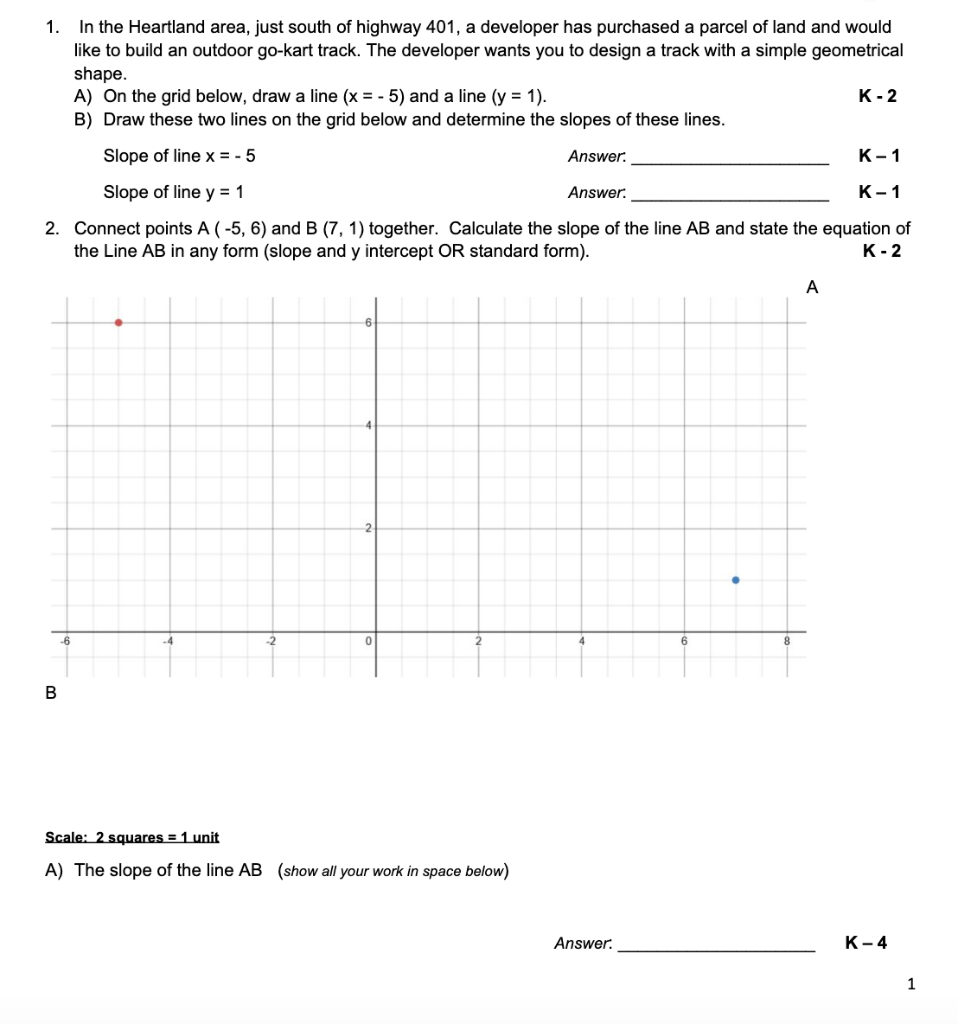1. In the Heartland area, just south of highway 401, a developer has purchased a parcel of land and would like to build an outdoor go-kart track. The developer wants you to design a track with a simple geometrical shape. A) On the grid below, draw a line (x = -5) and a line (y = 1). B) Draw these two lines on the grid below and determine the slopes of these lines. K-2 Slope of line x = -5 Answer. K-1 Slope of line y = 1 Answer: K-1 2. Connect points A (-5, 6) and B (7, 1) together. Calculate the slope of the line AB and state the equation of the Line AB in any form (slope and y intercept OR standard form). K-2 A -6 2 0 6 B Scale: 2 squares = 1 unit A) The slope of the line AB (show all your work in space below) Answer. K-4 1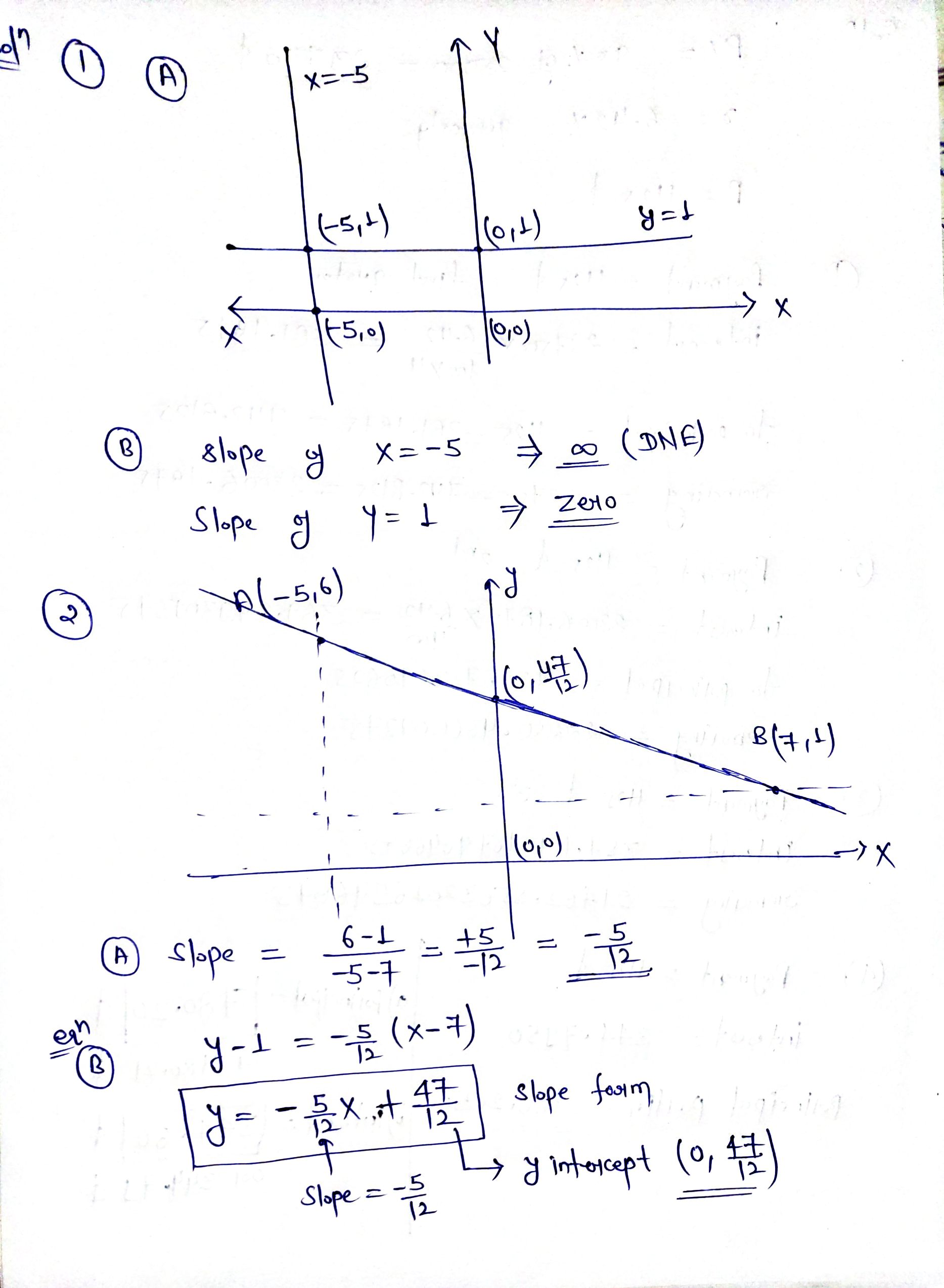##### Add Answer of: 1. In the Heartland area, just south of highway 401, a developer has purchased a parcel...
Similar Homework Help Questions
• ### MATH y = mx+b slope 1 In the Heartland area, just south of highway 401, a...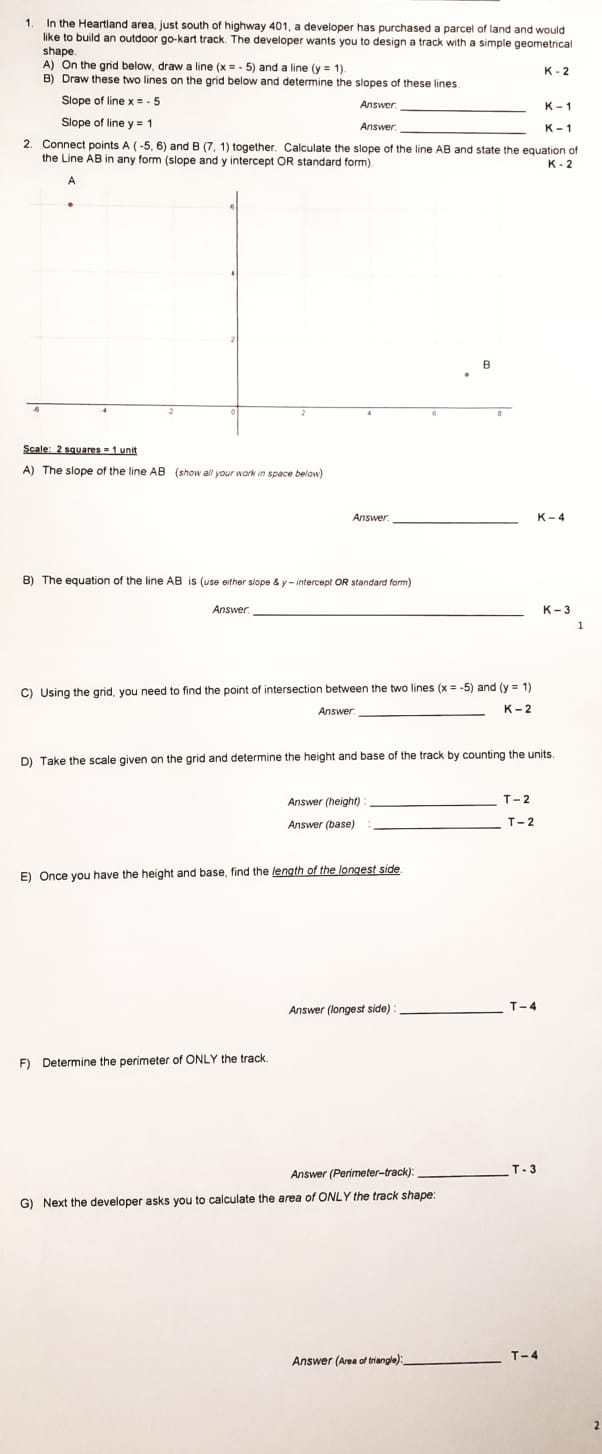MATH y = mx+b slope 1 In the Heartland area, just south of highway 401, a developer has purchased a parcel of land and would like to build an outdoor go-kart track. The developer wants you to design a track with a simple geometrical shape A) On the grid below, draw a line (x. 5) and a line (y = 1) K-2 B) Draw these two lines on the grid below and determine the slopes of these lines Slope of...

• ### MATH (SLOPE Y=MX+B) WORD PROBLEM 1 In the Heartland area, just south of highway 401, a...MATH (SLOPE Y=MX+B) WORD PROBLEM 1 In the Heartland area, just south of highway 401, a developer has purchased a parcel of land and would like to build an outdoor go-kart track. The developer wants you to design a track with a simple geometrical shape A) On the grid below, draw a line (x. 5) and a line (y = 1) K-2 B) Draw these two lines on the grid below and determine the slopes of these lines Slope of...

• ### MATH (SLOPE Y=MX+B) WORD PROBLEM (Please answer all questions) 1 In the Heartland area, just south...MATH (SLOPE Y=MX+B) WORD PROBLEM (Please answer all questions) 1 In the Heartland area, just south of highway 401, a developer has purchased a parcel of land and would like to build an outdoor go-kart track. The developer wants you to design a track with a simple geometrical shape A) On the grid below, draw a line (x. 5) and a line (y = 1) K-2 B) Draw these two lines on the grid below and determine the slopes of...

• ### Exercises M-6 Name 6 8 10 12 14 16 Determine the slopes of lines A and...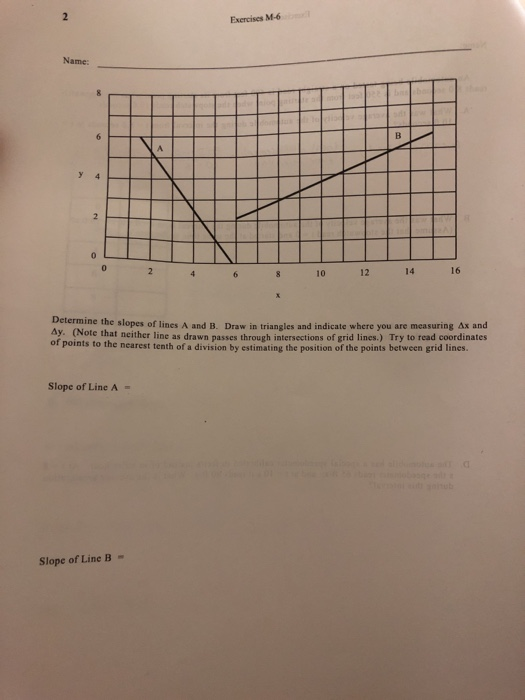Exercises M-6 Name 6 8 10 12 14 16 Determine the slopes of lines A and B Draw in triangles and indicate where you are measuring ax and Ay. (Note that neither line as drawn passes through intersections of grid lines.) Try to read coordinates of points to the nearest tenth of a division by estimating the position of the points between grid lines. Slope of Line A = Slope of Line B- Exercises M-6 Name Finding velocity from a...

• ### Problem 10.19 Consider the frame shown in (Figure 1). Assume the support at A is fixed...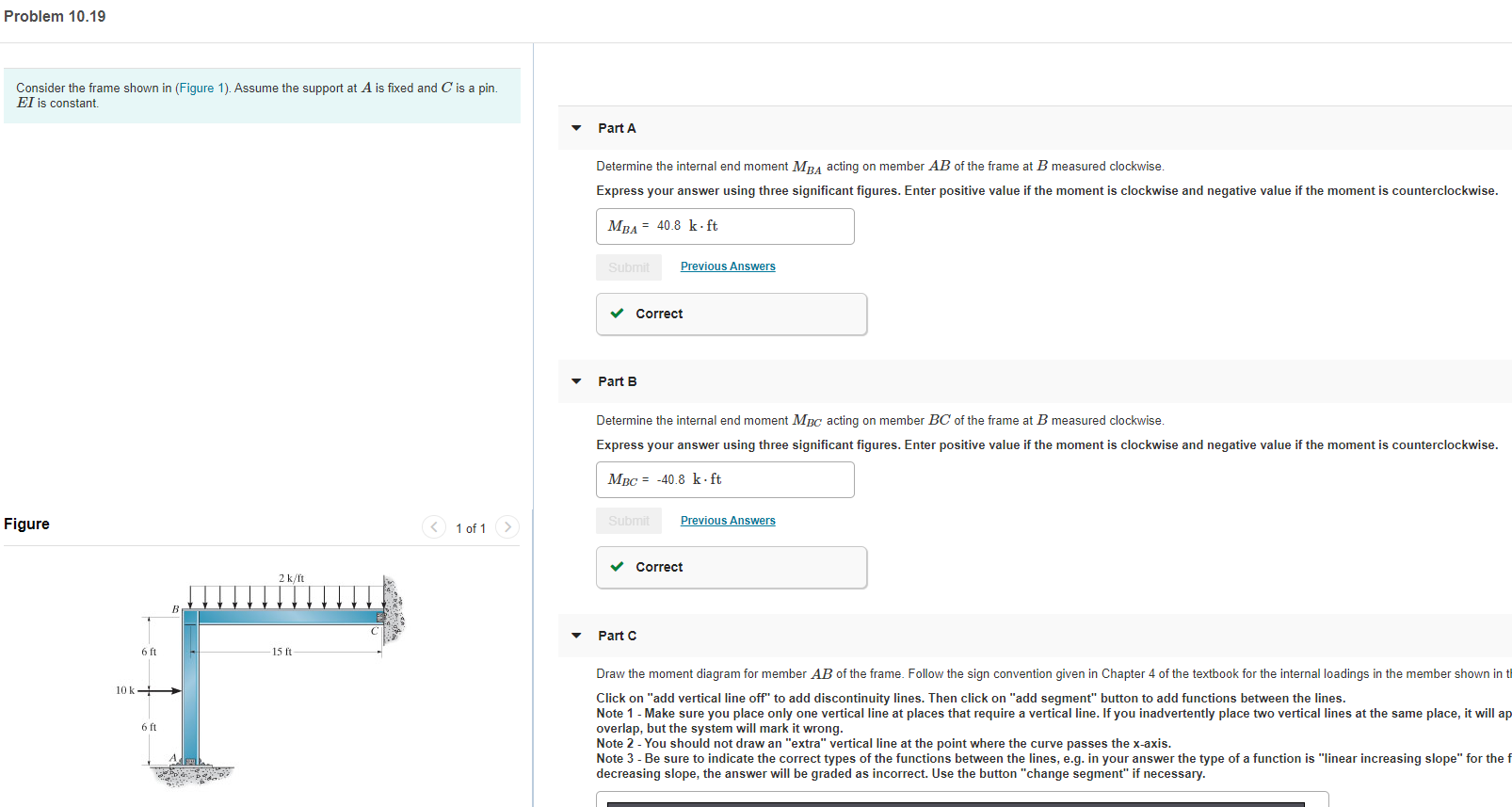Problem 10.19 Consider the frame shown in (Figure 1). Assume the support at A is fixed and C is a pin. EI is constant. Part A Determine the internal end moment MBA acting on member AB of the frame at B measured clockwise. Express your answer using three significant figures. Enter positive value if the moment is clockwise and negative value if the moment is counterclockwise. MBA = 40.8 k ft Submit Previous Answers Correct Part B Determine the internal...

• ### just slope

The experimental data in the table below define y as a function ofx.x012345y3.92.42.22.62.83.8A. Let P be the point (2, 2.2). Find the slopes of the secant linesPQ when Q is the point of the graph with x coordinate x1.x101345slopeB. Draw the graph of the function for yourself and estimate theslope of the tangent line at P.---------------------------------------------------------------------------------------------------------Q- 2A tank holds 800 gallons of water, which drains from thebottom of the tank in half an hour. The values in the table showthe...

• ### PART A Draw the shear diagram for the beam. Follow the sign convention. (Figure 1) Click on "add vertical line off" to add discontinuity lines. Then click on "add segment" button to a...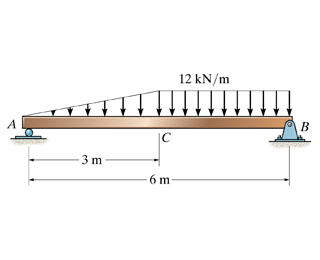PART A Draw the shear diagram for the beam. Follow the sign convention. (Figure 1) Click on "add vertical line off" to add discontinuity lines. Then click on "add segment" button to add functions between the lines. Note 1 - You should not draw an "extra" discontinuity line at the point where the curve passes the x-axis. Note 2 - The curve you choose from the drop-down is only a pictorial representation of a real quadratic/cubic curve. The equation of...

• ### Write the point slope form of the line's equation satisfying the given conditions. Then use the...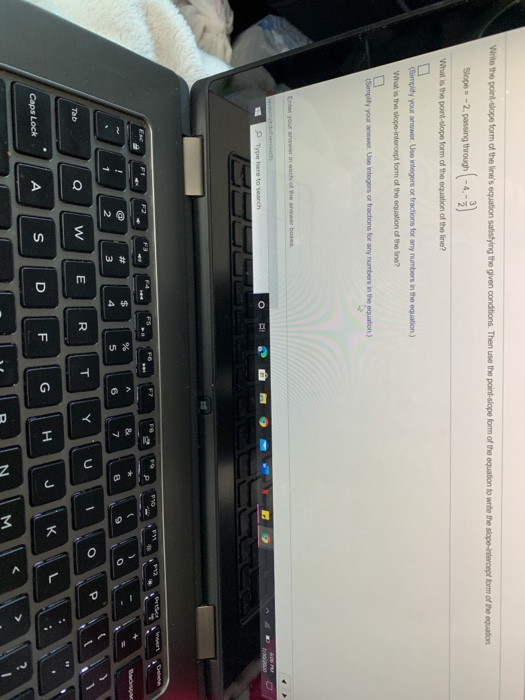Write the point slope form of the line's equation satisfying the given conditions. Then use the point slope form of the equation to write the slope-intercept form of the equation Slopes -2, passing through -4,- What is the point-slope form of the equation of the line? (Simplity your answer. Use integers or fractions for any numbers in the equation) What is the slope-intercept form of the equation of the line? (Simplify your wwwer. Ute integer or tractions for any numbers...

Justify your answer:1.) Which line is steeper?y= 4/3x - 5 or y= 6/5x - 12.) Does the slope of line AB exist?A (-2,7) B (-2,-1)

• ### Show that the point P(7,7) is on the perpendicular bisector of the line segment joining A (6,-1) to B (0, 3)

Show that the point P(7,7) is on the perpendicular bisector of the line segment joining A (6,-1) to B (0, 3). Also, verify that P is the same distance from A and B. For what values of k is the line y=l tangent to the circle (x-3)^2 + (y+2)^2 = 36? Thanks!Finished :D.Ok, for the first part, find the midpoint of the line AB. THen, find the slope . Now take the perpendicular slope to AB (the negative reciprocal). Now...

Free Homework App